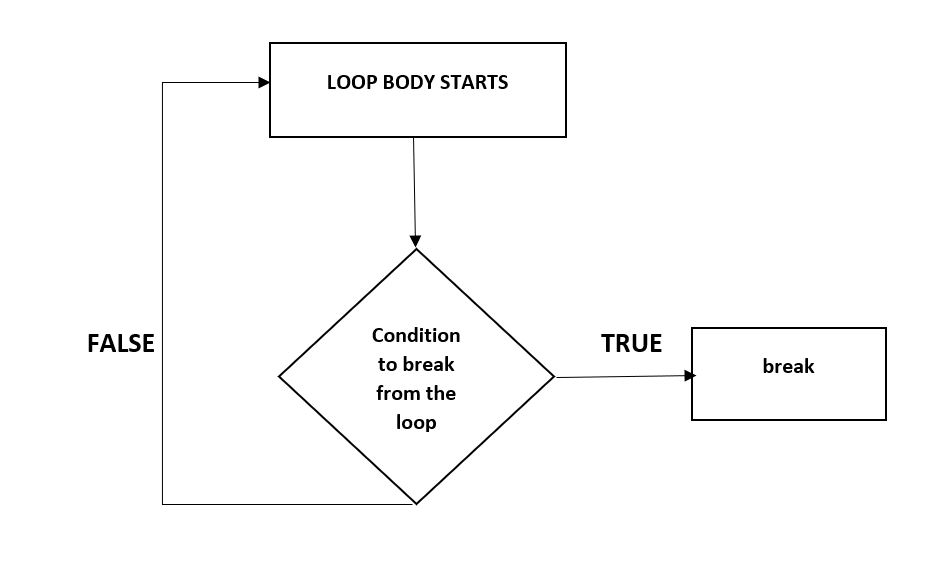×

Search anything:

# Break Statement in C

#### Software Engineering C break statementGet this book -> Problems on Array: For Interviews and Competitive Programming

Reading time: 20 minutes | Coding time: 2 minutes

Break Statement is a loop control statement which is used to terminate the loop or move program control to the code statement just after the loop. As soon as the break statement is encountered from within a loop, the loop iterations stops there and control returns from the loop immediately to the first statement after the loop.

Syntax : break; Always in small lettersBreak statements are used

1. When we are not sure about the actual number of iterations for the loop
2. We want to terminate the loop based on some condition.

We will see here the usage of break statement with three different types of loops:

• Simple loops
• Infinite loop
• Nested loop
• Switch case

### Simple loops

Consider the situation where we want to search an element in an array. To do this, use a loop to traverse the array starting from the first index and compare the array elements with the given key.
Below is the implementation of this idea:

``````// C program to illustrate simple loops
#include <stdio.h>

void findElement(int arr[], int size, int key)
{
// loop to traverse array and search for key
for (int i = 0; i < size; i++) {
if (arr[i] == key) {
printf("Element found at position: %d ",i + 1);
}
}
}

int main()
{
int arr[] = { 1, 2, 3, 4, 5, 6 };
int n = 6; // no of elements
int key = 3; // key to be searched
findElement(arr, n, key);
return 0;
}
``````

Output:

``````Element found at index: 3
``````

The above code runs fine with no errors. But the above code is not efficient. The above code completes all the iterations even after the element is found.

To avoid these useless iterations, we can use break statement in our program. Once the break statement is encountered the control from loop will return immediately after condition gets satisfied. So will use the break statement with the if condition which compares the key with array elements as shown below:

``````// C program to illustrate simple loops
#include <stdio.h>

void findElement(int arr[], int size, int key)
{
// loop to traverse array and search for key
for (int i = 0; i < size; i++) {
if (arr[i] == key) {
printf("Element found at position: %d ",i + 1);
==using break to terminate loop execution==
break;
}
}
}

// Driver program to test above function
int main()
{
int arr[] = { 1, 2, 3, 4, 5, 6 };
int n = 6; // no of elements
int key = 3; // key to be searched
findElement(arr, n, key);
return 0;
}
``````

### Infinite loops :

break statement can be included in an infinite loop with a condition in order to terminate the execution of the infinite loop.
Consider the below infinite loop:

``````// C program to illustrate
// using break statement

#include <stdio.h>
int main()
{
int i = 1;
while (1) {
printf("%d",i);
i++;
}
return 0;
}
``````

Note: Please don't run the above program in your compiler as it is an infinite loop so you may have to forcefully exit the compiler to terminate the program.

In the above program the loop condition based on which the loop terminates is always true. So, the loop executes infinite number of times. We can correct this using break statement as shown below:

``````// C program to illustrate
// using break statement

#include <stdio.h>
int main()
{
int i = 1;
while (1) {
if (i > 10)
break;
printf("%d",i);
i++;
}
return 0;
}
``````

Output:

``````1 2 3 4 5 6 7 8 9 10
``````

The above code restricts the number of loop iterations to 10.

### Nested loops:

We can also use break statement while working with nested loops. If the break statement is used in the innermost loop. The control will come out only from the innermost loop. Below is the example of using break with nested loops:

``````// C program to illustrate
// using break statement

#include <stdio.h>
int main()
{
int i,j;
for (i = 0; i < 5; i++) {
for (j = 1; j <= 10; j++) {
if (j > 3)
break;
else
printf("*");
}
printf("\n");
}

return 0;
}
``````

Output:

``````***
***
***
***
***
``````

Note : break applies to only the loop within which it is present.

### Switch case :

The break statement is used inside the switch to terminate a statement sequence. When a break statement is reached, the switch terminates, and the flow of control jumps to the next line following the switch statement.
The break statement is optional. If omitted, execution will continue on into the next case. The flow of control will fall through to subsequent cases until a break is reached.Below is the example of using break with switch case:

``````// C program to illustrate
// using break statement
#include <stdio.h>
int main()
{
int x = 2;
switch (x)
{
case 1: printf("Choice is 1");
break;
case 2: printf("Choice is 2");
break;
case 3: printf("Choice is 3");
break;
default: printf("Choice other than 1, 2 and 3");
break;
}
return 0;
}
``````

Output:

``````Choice is 2
``````

### Question

#### The keyword ‘break’ cannot be simply used within _________

if-else
while
do-while
switch case
If we use break statement within if else then the else condition will not be checked and hence the condition will not be checked completely in such case we use goto statements.#### Harshita Sahai

Maintainer at OpenGenus | Previously Software Developer, Intern at OpenGenus (June to August 2019) | B.Tech in Information Technology from Guru Gobind Singh Indraprastha University (2017 to 2021)

Break Statement in C